## Math 501: Differential Geometry

Homework 8 <me@tylerlogic.com>

April 14, 2013
http://coursework.tylerlogic.com/courses/math501/homework08

1 do Carmo pg 237 problem 1

With F = 0, the Christoffel symbols simplify to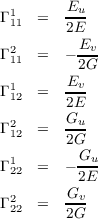From here, one would use the equation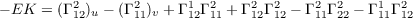and expand/simplify appropriately to get the desired answer. However, I was not able to find the correct sequence of expansions/simplifications of the equation resulting from the combination of the above equations.

2 do Carmo pg 237 problem 2

For E = G = λ(u,v) and F = 0 we can use the previous problem to find K to be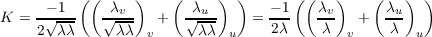Thus since Δlog λ =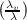v +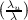u then we have that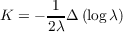Now let λ = (u2 + v2 + c)-2. To make the following computation easier to follow, we’ll define γ = u2 + v2 + c, i.e. λ = γ-2. We have the following value of K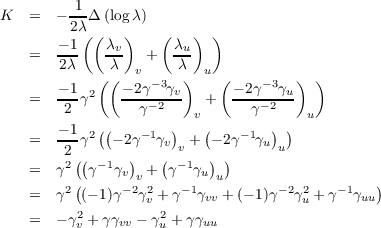With our definition of γ, γu = 4u, γv = 4v, and γuu = γvv = 4. Continuing the equation above we obtain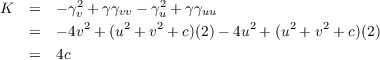3

Let S be a surface with geodesic coordinates X so that E = 1 and F = 0.

(a) Christoffel Symbols

Since E = 1 then Eu = Ev = 0 and thus with F = 0 most of the Christoffel symbols are zero. Making use of the equations in (2) on pg 232 of do Carmo, we have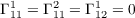as well as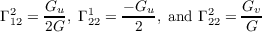(b) Gaussian Curvature

From the equations of the previous part of the problem, due to most of the Christoffel Symbols being zero, we have this small equation for the Gaussian curvature.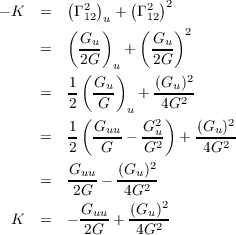(c) g for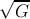Let g =. Then G = g2, Gu = 2ggu and Guu = 2gu2 + 2gguu, so substituting these equations into the result of the previous part of the problem gets us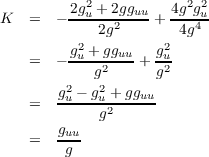4

(a)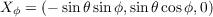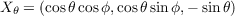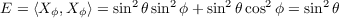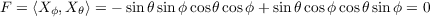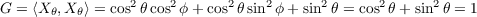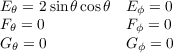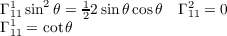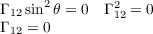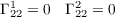(b)

Let W(s) = a(s)Xθ + b(s)Xϕ. With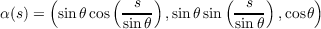we have u= -sin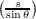. Thus by equation (1) on page 239 of do Carmo, we have the following since all Christoffel Symbols are zero except for Γ111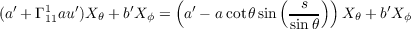(c)

Solving the ODEs of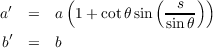we find that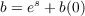and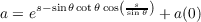How much does the parallel transport of a vector rotate after one loop? ???

5

With the definition of α above we get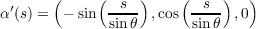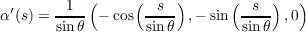Curvature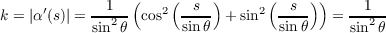Normal Curvature Let N be the normal map on the sphere N(p) = p. Than at some p in the trace of α we have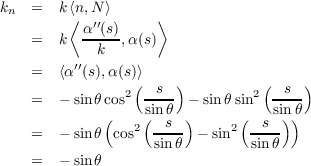Geodesic Curvature We have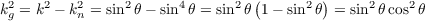6

(a) Non-length-minimizing positive curvature geodesic

The sketch in Figure 1 has a geodesic between p and q that is not length minimizing. The curve that travels “the long way” along the great circle containing p and q will be a geodesic, but not length-minimizing. The geodesic traveling “the short way” will be length-minimizing though.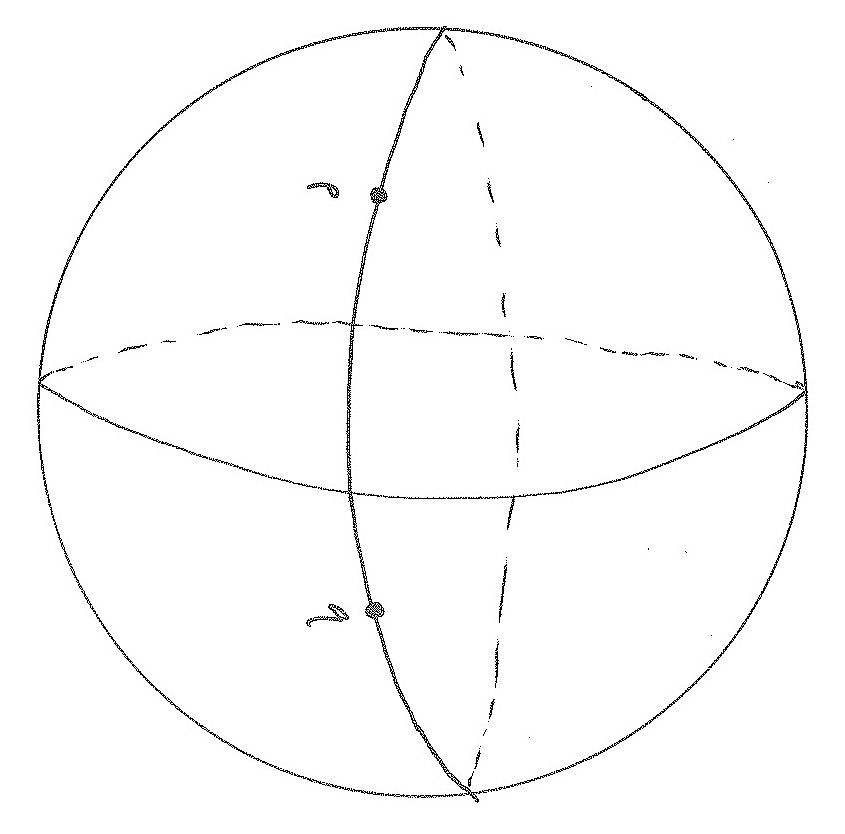Figure 1: Sketch of positive curvature surface with a geodesic between p and q which is not length-minimizing.

(b) Non-length-minimizing zero curvature geodesic

The sketch in Figure 2 has a geodesic between p and q that is not length minimizing. Again going the long way, but this time along a horizontal circle of this cylinder.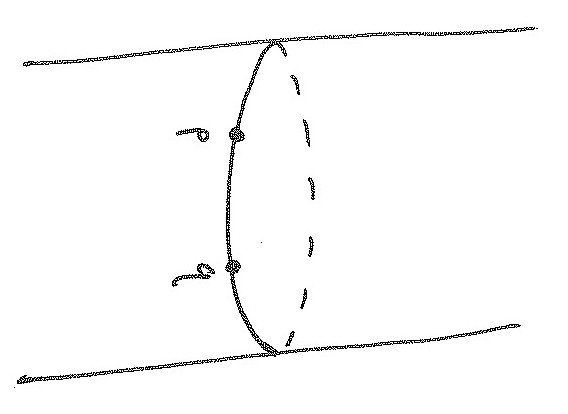Figure 2: Sketch of zero curvature surface with a geodesic between p and q which is not length-minimizing.

(c) Non-length-minimizing negative curvature geodesic

The sketch in Figure 3 has a geodesic between p and q that is not length minimizing. Again going the long way, but this time around the “waist” of this surface of revolution.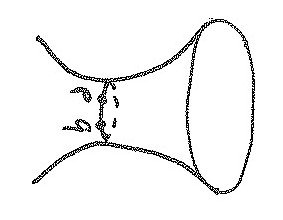Figure 3: Sketch of negative curvature surface with a geodesic between p and q which is not length-minimizing.

(d)

(e) A surface where any two points can be joined by a geodesic, but the geodesic is only defined for a finite amount of time.

The sketch in Figure 4 has a geodesic between every two points, namely the straight line between them. However, since geodesics need to have constant velocity, then only finite time geodesics could exist.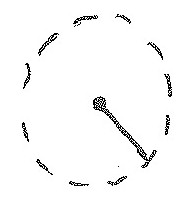Figure 4: Sketch of surface with only finite time geodesics.